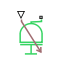# Variable Inertia

Time-varying inertia specified as physical signal input

## Library

•## Description

This block models the effects of a time-varying inertia. Components with variable inertia include satellites unfolding arrays of solar panels. A physical signal input port provides the instantaneous inertia at each time step throughout simulation. To minimize solver errors, avoid discontinuities and abrupt changes in the input signal.

As the component inertia changes, so does the inertial torque acting on that component:

`${T}_{I}=I\stackrel{˙}{\omega },$`

where:

• TI is the inertial torque.

• I is the variable inertia.

• ω is the angular velocity of the inertia.

The physical signal input saturates at the minimum inertia specified in the block property inspector. Whenever the input falls below the minimum inertia, the block performs its calculations on the minimum inertia instead. The inertial torque becomes:

`${T}_{I}={I}_{Min}\stackrel{˙}{\omega },$`

where:

• IMin is the minimum inertia allowed.

The angular momentum of the variable inertia is conserved. In the absence of external torques, a decrease in the moment of inertia leads to a proportional increase in angular velocity. The angular momentum remains constant until a net external torque causes it to change.

### Variables

To set the priority and initial target values for the block variables prior to simulation, use the Initial Targets section in the block dialog box or Property Inspector. For more information, see Set Priority and Initial Target for Block Variables and Initial Conditions for Blocks with Finite Moist Air Volume.

Nominal values provide a way to specify the expected magnitude of a variable in a model. Using system scaling based on nominal values increases the simulation robustness. Nominal values can come from different sources, one of which is the Nominal Values section in the block dialog box or Property Inspector. For more information, see Modify Nominal Values for a Block Variable.

## Ports

The block has one conserving rotational port and one physical signal input port. The conserving rotational port identifies the driveline component whose inertia varies with time. The physical signal input port provides the means to specify the inertia as a function of time.

## Parameters

Minimum inertia

Lowest value that the variable inertia can take on during simulation. The physical signal input saturates at this value. If the input falls below the minimum inertia, it is set equal to the minimum inertia value. The default value is `0.1` `kg*m^2`.

## Version History

Introduced in R2015b